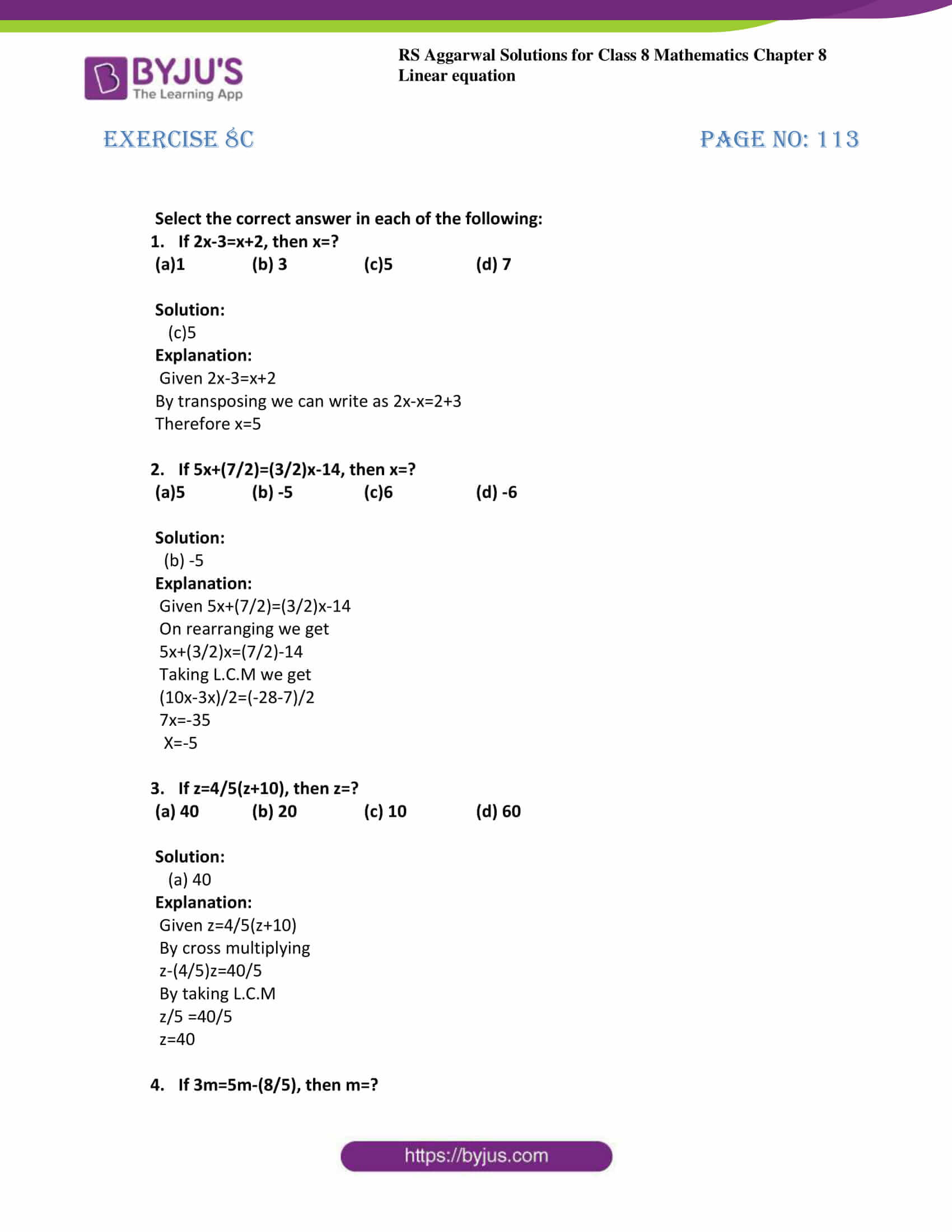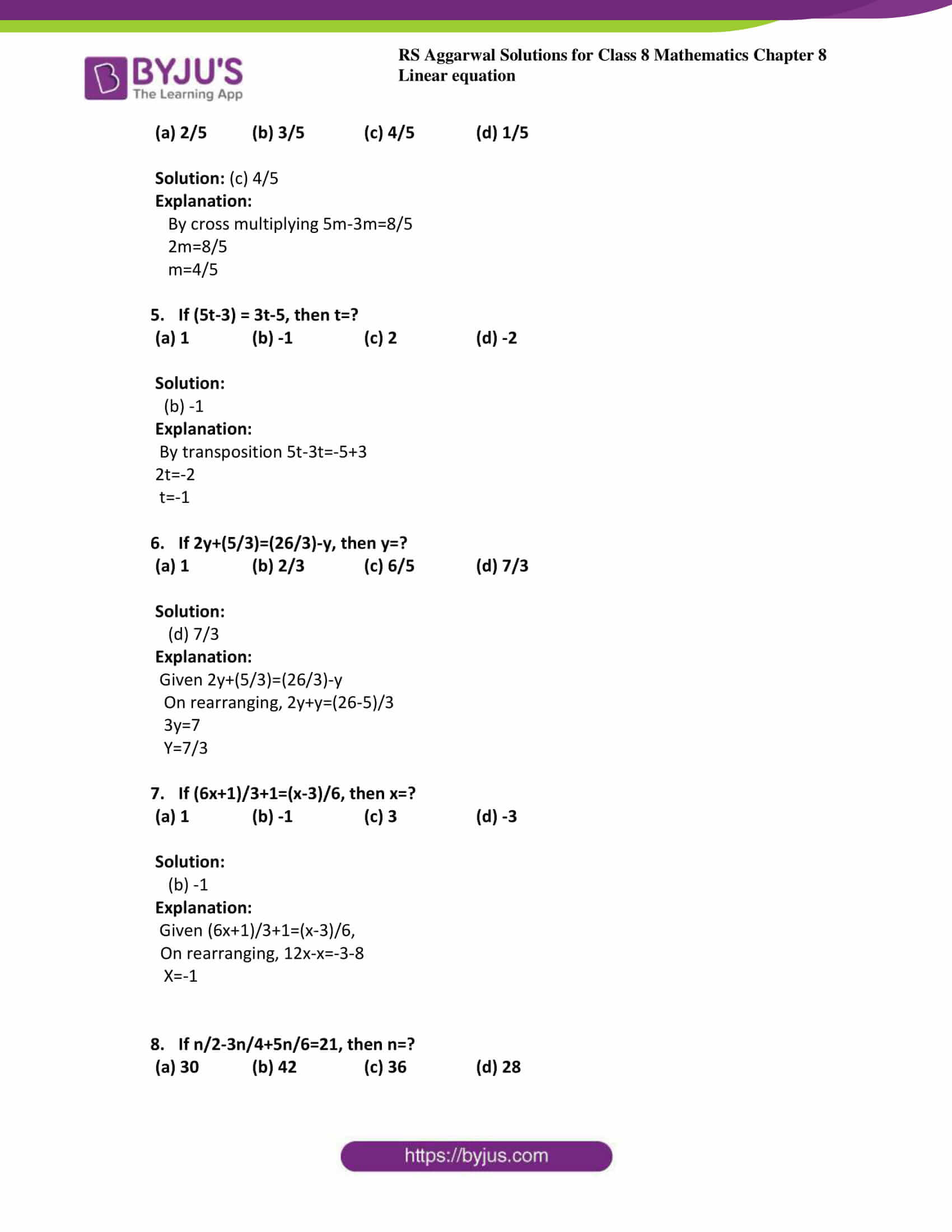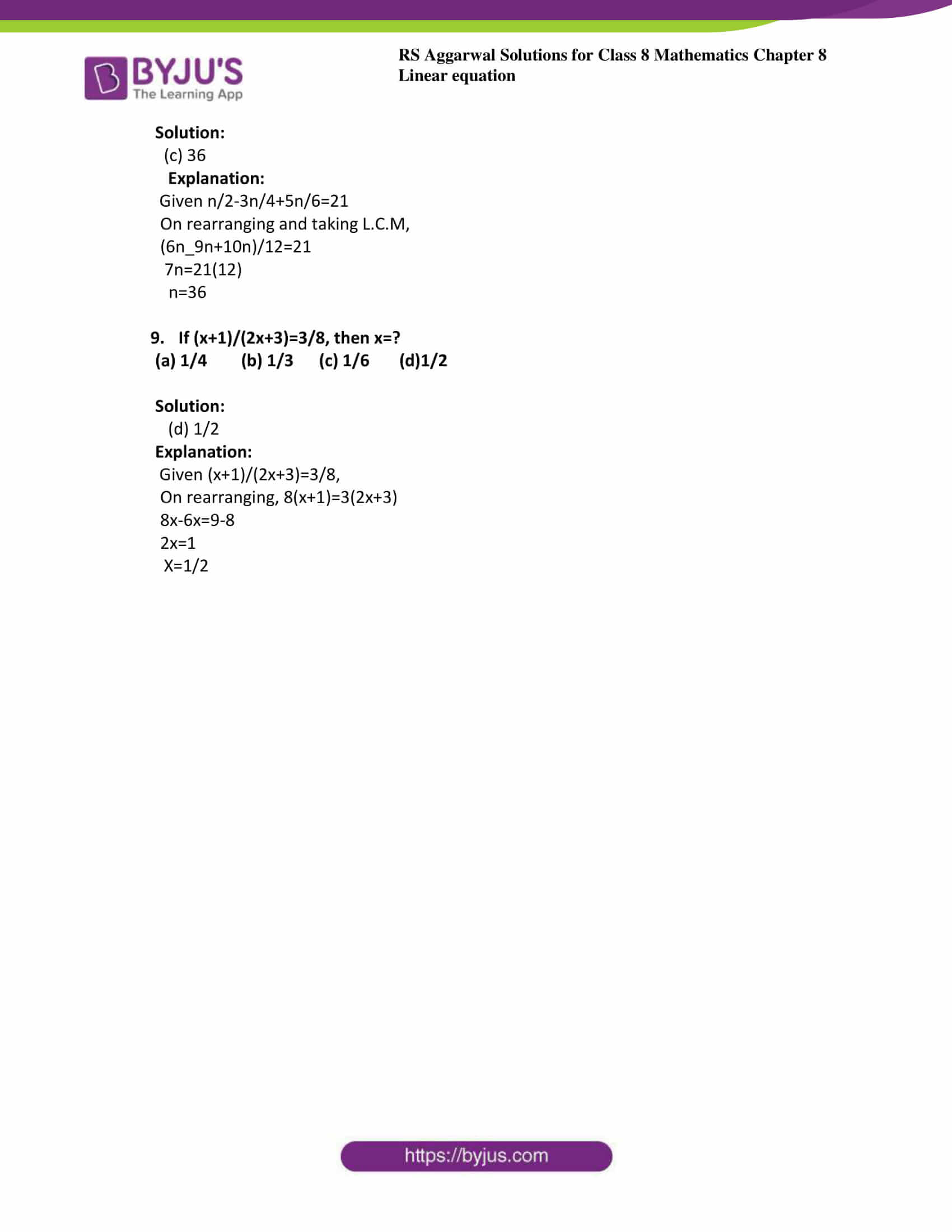# RS Aggarwal Solutions for Class 8 Maths Chapter 8 - Linear Equations Exercise 8C

RS Aggarwal Solutions for the Exercise 8C of Class 8 Maths Chapter 8, Linear Equations pdf is available here. By practising regularly, the students can obtain reliable results in the Class 8 exams by using the RS Aggarwal Solutions. BYJU’S experts solve the given exercise in such a way that students can understand easily. This exercise contains an objective type of questions so that students can obtain an application level of knowledge. And, by this kind of problems, they also get to know the value of time.

By practising the RS Aggarwal Solutions for class 8, students will be able to perceive the concepts entirely. Hence, students whose aim is to score high in the examinations are advised to go through RS Aggarwal Solutions for Class 8.

## Download PDF of RS Aggarwal Solutions for Class 8 Maths Chapter 8 – Linear Equations Exercise 8C### Access answers to Maths RS Aggarwal Solutions for Class 8 Chapter 8 – Linear Equations Exercise 8C

Select the correct answer in each of the following:

1. If 2x-3=x+2, then x=?

(a)1 (b) 3 (c)5 (d) 7

Solution:

(c)5

Explanation:

Given 2x-3=x+2

By transposing we can write as 2x-x=2+3

Therefore x=5

2. If 5x+(7/2)=(3/2)x-14, then x=?

(a)5 (b) -5 (c)6 (d) -6

Solution:

(b) -5

Explanation:

Given 5x+(7/2)=(3/2)x-14

On rearranging we get

5x+(3/2)x=(7/2)-14

Taking L.C.M we get

(10x-3x)/2=(-28-7)/2

7x=-35

x=-5

3. If z=4/5(z+10), then z=?

(a) 40 (b) 20 (c) 10 (d) 60

Solution:

(a) 40

Explanation:

Given z=4/5(z+10)

By cross multiplying

z-(4/5)z=40/5

By taking L.C.M

z/5 =40/5

z=40

4. If 3m=5m-(8/5), then m=?

(a) 2/5 (b) 3/5 (c) 4/5 (d) 1/5

Solution: (c) 4/5

Explanation:

By cross multiplying 5m-3m=8/5

2m=8/5

m=4/5

5. If (5t-3) = 3t-5, then t=?

(a) 1 (b) -1 (c) 2 (d) -2

Solution:

(b) -1

Explanation:

By transposition 5t-3t=-5+3

2t=-2

t=-1

6. If 2y+(5/3)=(26/3)-y, then y=?

(a) 1 (b) 2/3 (c) 6/5 (d) 7/3

Solution:

(d) 7/3

Explanation:

Given 2y+(5/3)=(26/3)-y

On rearranging, 2y+y=(26-5)/3

3y=7

y=7/3

7. If (6x+1)/3+1=(x-3)/6, then x=?

(a) 1 (b) -1 (c) 3 (d) -3

Solution:

(b) -1

Explanation:

Given (6x+1)/3+1=(x-3)/6,

On rearranging, 12x-x=-3-8

x=-1

8. If n/2-3n/4+5n/6=21, then n=?

(a) 30 (b) 42 (c) 36 (d) 28

Solution:

(c) 36

Explanation:

Given n/2-3n/4+5n/6=21

On rearranging and taking L.C.M,

(6n_9n+10n)/12=21

7n=21(12)

n=36

9. If (x+1)/(2x+3)=3/8, then x=?

(a) 1/4 (b) 1/3 (c) 1/6 (d)1/2

Solution:

(d) 1/2

Explanation:

Given (x+1)/(2x+3)=3/8,

On rearranging, 8(x+1)=3(2x+3)

8x-6x=9-8

2x=1

x=1/2

Exercise 8A

Exercise 8B

## RS Aggarwal Solutions for Class 8 Maths Chapter 8 – Linear Equations Exercise 8C

Exercise 8C of RS Aggarwal Solutions for Chapter 8, Linear Equations covers all the topics related to equations and rules for solving a linear equation. Some of the topics focused prior to exercise 8C include the following.

• Definition of equation
• Rules for solving a linear equation
• Transposing
• Cross multiplication
• Applications of linear equations

The RS Aggarwal Solutions can help the students in practising and learning each and every concept as it provides solutions to all questions asked in RS Aggarwal textbook.